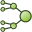# FM Card

The FM card is used to instruct the Feko solver to calculate the solution using accelerated methods, for example, using the multilevel fast multipole method (MLFMM) or adaptive cross-approximation (ACA). An option is available to apply compression to looped plane wave sources.

On the Solve/Run tab, in the Solution settings group, click theMLFMM (FM) icon.

## Parameters:

None
Neither the MLFMM nor the ACA is activated.
Use マルチレベル高速多重極法 (MLFMM)
The MLFMM is used instead of the standard モーメント法 (MoM) to calculate the solution on all structures.
The ACA method is used to calculate the solution. This method approximates the MoM impedance matrix by constructing a sparse H-matrix (only a few selected elements are computed). This method is applicable to low frequency problems or when using a special Green’s function.
Activate additional stabilisation for the MLFMM to address models with severe convergence problems.
Box size at finest level
The MLFMM is based on a hierarchical tree-based grouping algorithm, and depending on the frequency and the model dimensions Feko automatically determines the number of levels in this tree and the size of the boxes at the finest level. It is also recommended that this default box size of 0.23λ is kept. When there is no convergence in the MLFMM, then advanced users might try to slightly increase or decrease this box size by setting it manually (the input is in terms of the wavelength).
Field calculation methods
The ソルバー determines automatically which calculation method to use for the fastest field calculation.
Enable fast far field calculation
If selected, the MLFMM method is included in the available options for calculating the far field (default). If not selected, the ソルバー uses either traditional integration or other fast methods to calculate the far field.
Enable fast near field calculation
If selected, the MLFMM method is included in the available options for calculating the near field (default). If not selected, the ソルバー uses either traditional integration or other fast methods to calculate the near field.
Compression for looped plane wave sources

Compression of a plane wave loop over direction of incidence can be used to speed up calculations over multiple directions of incidence (for example, when calculating RCS).

Auto
The ソルバー determines automatically if compression should be used for the model (if the method is likely to speed up the solution).
Enable compression
Enable compression for looped plane wave sources in the model.
Disable compression
Do not use compression for looped plane wave sources in the model.

## MLFMM

Note: The MLFMM is not supported in conjunction with the multilayer Green's function.